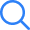# Power Systems T...

TIME LEFT -
• ###### Question 1
2 / -0.33

Increased fuel cost in Rs/Mwh for a plant consisting of units are given by

$$\frac{{d{F_1}}}{{d{P_1}}} = 0.4{P_1} + 400$$

$$\frac{{d{F_2}}}{{d{P_2}}}= 0.48{P_2} + 320$$

The allocation of load P1 and P2 between the unit 1 and unit 2, respectively, for the minimum cost of generation for a total load of 900 MW is

• ###### Question 2
2 / -0.33

A short three phase short transmission line in phase co-ordinates is represented by a series impedance matrix as $$\left[ {\begin{array}{*{20}{c}}{{Z_s}}&{{Z_m}}&{{Z_m}}\\{{Z_m}}&{{Z_s}}&{{Z_m}}\\{{Z_m}}&{{Z_m}}&{{Z_m}}\end{array}} \right]$$

If the negative sequence impedance is (2 + 9) Ω and zero sequence impedance is (8 + j42) Ω, which of the following is/are true?

• ###### Question 3
2 / -0.33

A 100 × 100 bus admittance matrix for an electric power system has 600 non-zero elements. Which of the following is/are true?

• ###### Question 4
2 / -0.33

The initial value of DC offset in a three-phase fault depends on:

• ###### Question 5
2 / -0.33

Which of the following has a problem in convergence for a system with long radial lines?

• ###### Question 6
2 / -0.33

The positive, negative and zero sequence impedances of 3-phase synchronous generator are j 0.5 pu, j 0.3 pu and j 0.2 pu respectively. When the symmetrical fault occurs on the machine terminals. Find the fault current. The generator neutral is grounded through reactance of j0.1 pu

• ###### Question 7
2 / -0.33

A 100 MVA synchronous generator operates on full load at a frequency of 50 Hz. Inertia constant is 8 MJ/MVA. The load is suddenly reduced 100 MW. Due to time lag in governor system, the steam valve begins to close after 0.4 seconds. The change in frequency that occurs in this time is_____

• ###### Question 8
2 / -0.33

In a 132 kV, 50 Hz system, reactance and capacitance up to the location of the circuit breaker is 5 Ω and 0.02 μF respectively. A resistance of 500 Ω is connected across the breaker of the circuit breaker. The damped frequency of oscillation is ______ (in kHz)

• ###### Question 9
2 / -0.33

A circuit breaker is employed to quench the magnetizing current of a 80 MVA transformer at 230 kV. The magnetizing current is 4% of full load current. Now, the circuit breaker is opened when the magnetizing current is as its peak value. The stray capacitance is 4 pF and inductance is 25 mH. The maximum voltage appears across the circuit is ______(in kV)

• ###### Question 10
2 / -0.33

The power system has two generating plants and power being dispatched economically with P1 = 150 MW and P2 = 200 MW. The loss co-efficient are B11 = 0.003 MW-2, B22 = 0.001 MW-2 and B12 = - 0.0004 MW-2. The penalty factor for the plant 1 will be _________ (up to two decimal places)

• ###### Question 11
2 / -0.33

The sending and voltage of a three phase 50 Hz lossless transmission line is maintained at 400 kV. The inductance and capacitance of the line are 8 mH/km/phase and 200 nF/ km/ Phase respectively.

To maintain a voltage of 400 kV at receiving end, when the line is delivering 600 MW load, which of the following is/are true?

• ###### Question 12
2 / -0.33

A balanced 3ϕ load of 30 MW is supplied at 132 kV, 50 Hz & 0.85 pf lagging by means of transmission line. The series impedance of single conductor is (20 + j52) Ω and total phase-neutral admittance is 315 × 10-6 mho. For a nominal T-method, which of the following is / are true regarding the A, B, C, D constants of the line.

• ###### Question 13
2 / -0.33

A cylindrical rotor synchronous generator is operating at a terminal voltage of 1 pu with an internal emf of (1 + j0.5) pu. It has a steady state synchronous reactance of 0.8 pu and sub transient reactance of 0.2 pu.

If there is a three-phase solid short circuit fault at the terminal of the generator, which of the following is/are true?

• ###### Question 14
2 / -0.33

A cable is graded with three dielectrics of permittivities 4, 3 and 2. The maximum permissible potential gradient for all dielectrics is same and equal to 30 kV / cm. The core diameter is 1.5 cm and sheath diameter is 5.5 cm the rms value of working voltage is ______ (in kV)

• ###### Question 15
2 / -0.33

A 3ϕ 50 Hz 320 km long transmission line has inductance of 1.98 mH / km / phase & C = 9.93 × 10-9 F / km. The line supplies a load of 75 MVA, 0.8 pf lagging at 400 kV. The MVAR rating of 3ϕ shunt reactor to be installed at the receiving end to sending end voltage at rated value is

• ###### Question 16
2 / -0.33

A 50 Hz, a synchronous generator capable to supply 400 MW connected to a large power system and delivering 80 MW when a three-phase fault occurs at its terminals. If the maximum power angle is to be 85°, the time in which the fault must be cleared is _____ (in sec)

(Assume the base value is 100 MW  and consider the inertia constant H = 7 MJ / MVA )

Submit Test##### Question Analysis
• Answered - 0

• Unanswered - 16

• 1
• 2
• 3
• 4
• 5
• 6
• 7
• 8
• 9
• 10
• 11
• 12
• 13
• 14
• 15
• 16
Submit Test×
Open Now